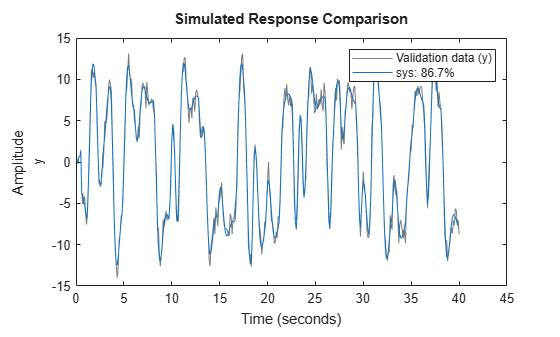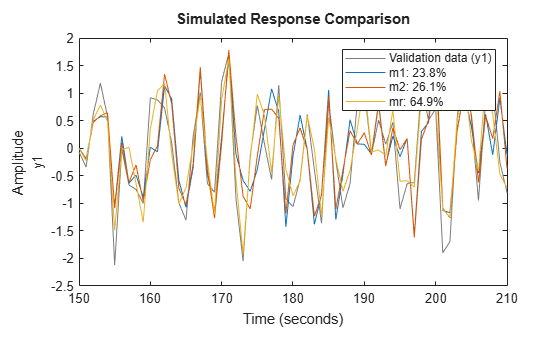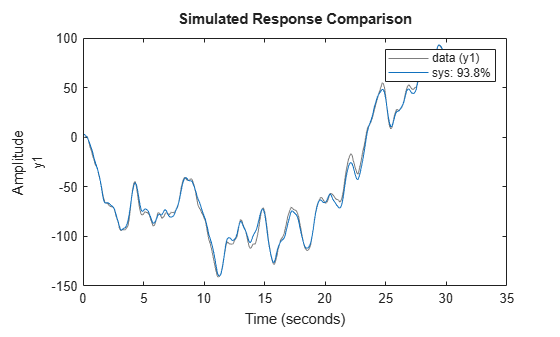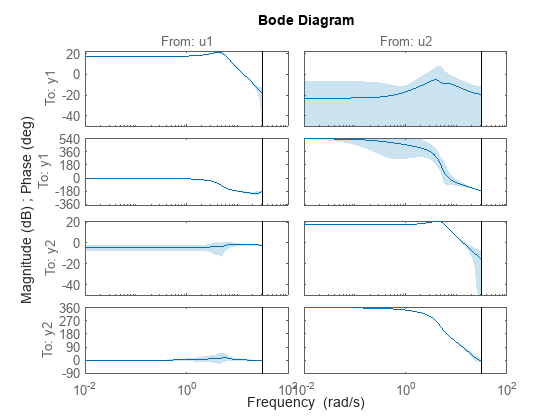# polyest

Estimate polynomial model using time- or frequency-domain data

## Syntax

```sys = polyest(tt,[na nb nc nd nf nk]) sys = polyest(u,y,[na nb nc nd nf nk]) sys = polyest(data,[na nb nc nd nf nk]) sys = polyest(___,Name,Value) sys = polyest(tt,init_sys) sys = polyest(u,y,init_sys) sys = polyest(tt,init_sys) sys = polyest(___, opt) [sys,ic] = polyest(___) ```

## Description

`sys = polyest(tt,[na nb nc nd nf nk])` estimates a polynomial model `sys` using the data contained in the variables of timetable `tt`. The software uses the first Nu variables as inputs and the next Ny variables as outputs, where Nu and Ny are determined from the dimensions of the specified polynomial orders.

`sys` is of the form

`$A\left(q\right)y\left(t\right)=\frac{B\left(q\right)}{F\left(q\right)}u\left(t-nk\right)+\frac{C\left(q\right)}{D\left(q\right)}e\left(t\right)$`

A(q), B(q), F(q), C(q) and D(q) are polynomial matrices. u(t) is the input, and `nk` is the input delay. y(t) is the output and e(t) is the disturbance signal. `na` ,`nb`, `nc`, `nd` and `nf` are the orders of the A(q), B(q), C(q), D(q) and F(q) polynomials, respectively.

To select specific input and output channels from `tt`, use name-value syntax to set `'InputName'` and `'OutputName'` to the corresponding timetable variable names.

`sys = polyest(u,y,[na nb nc nd nf nk])` uses the time-domain input and output signals in the comma-separated matrices `u`,`y`. The software assumes that the data sample time is 1 second. To change the sample time, set `Ts` using name-value syntax.

`sys = polyest(data,[na nb nc nd nf nk])` uses the time-domain or frequency-domain data in the data object `data`.

`sys = polyest(___,Name,Value)` estimates a polynomial model with additional attributes of the estimated model structure specified by one or more `Name,Value` arguments. You can use this syntax with any of the previous input-argument combinations.

`sys = polyest(tt,init_sys)` estimates a polynomial model using the linear system `init_sys` to configure the initial parameterization for estimation using the timetable `tt`.

`sys = polyest(u,y,init_sys)` uses the matrix data `u`,`y` for estimation..

`sys = polyest(tt,init_sys)` uses the data object `data`, for estimation.

`sys = polyest(___, opt)` estimates a polynomial model using the option set, `opt`, to specify estimation behavior.

`[sys,ic] = polyest(___)` returns the estimated initial conditions as an `initialCondition` object. Use this syntax if you plan to simulate or predict the model response using the same estimation input data and then compare the response with the same estimation output data. Incorporating the initial conditions yields a better match during the first part of the simulation.

## Input Arguments

 `tt` Estimation data, specified as a `timetable` that uses a regularly spaced time vector. `tt` contains variables representing input and output channels. For multiexperiment data, `tt` is a cell array of timetables of length `Ne`, where `Ne` is the number of experiments The software determines the number of input and output channels to use for estimation from the dimensions of the specified polynomial orders. The input/output channel selection depends on whether the `'InputName'` and `'OutputName'` name-value arguments are specified. If `'InputName'` and `'OutputName'` are not specified, then the software uses the first Nu variables of `tt` as inputs and the next Ny variables of `tt` as outputs.If `'InputName'` and `'OutputName'` are specified, then the software uses the specified variables. The number of specified input and output names must be consistent with Nu and Ny.For functions that can estimate a time series model, where there are no inputs, `'InputName'` does not need to be specified. For more information about working with estimation data types, see Data Domains and Data Types in System Identification Toolbox. `u`, `y` Estimation data, specified for SISO systems as a comma-separated pair of Ns-by-1 real-valued matrices that contain uniformly sampled input and output time-domain signal values. Here, Ns is the number of samples. For MIMO systems, specify `u`,`y` as an input/output matrix pair with the following dimensions: `u` — Ns-by-Nu, where Nu is the number of inputs.`y` — Ns-by-Ny, where Ny is the number of outputs. For multiexperiment data, specify `u`,`y` as a pair of 1-by-Ne cell arrays, where Ne is the number of experiments. The sample times of all the experiments must match. For time series data, which contains only outputs and no inputs, specify `y` only. For more information about working with estimation data types, see Data Domains and Data Types in System Identification Toolbox. `data` Estimation data. For time-domain estimation, `data` is an `iddata` object containing the input and output signal values. You can estimate only discrete-time models using time-domain data. For estimating continuous-time models using time-domain data, see `tfest`. For frequency-domain estimation, `data` can be one of the following: Recorded frequency response data (`frd` (Control System Toolbox) or `idfrd`) `iddata` object with its properties specified as follows:`InputData` — Fourier transform of the input signal`OutputData` — Fourier transform of the output signal`Domain` — ‘Frequency’It may be more convenient to use `oe` or `tfest` to estimate a model for frequency-domain data. `na` Order of the polynomial A(q). `na` is an Ny-by-Ny matrix of nonnegative integers. Ny is the number of outputs, and Nu is the number of inputs. `na` must be zero if you are estimating a model using frequency-domain data. `nb` Order of the polynomial B(q) + 1. `nb` is an Ny-by-Nu matrix of nonnegative integers. Ny is the number of outputs, and Nu is the number of inputs. `nc` Order of the polynomial C(q). `nc` is a column vector of nonnegative integers of length Ny. Ny is the number of outputs. `nc` must be zero if you are estimating a model using frequency-domain data. `nd` Order of the polynomial D(q). `nd` is a column vector of nonnegative integers of length Ny. Ny is the number of outputs. `nd` must be zero if you are estimating a model using frequency-domain data. `nf` Order of the polynomial F(q). `nf` is an Ny-by-Nu matrix of nonnegative integers. Ny is the number of outputs, and Nu is the number of inputs. `nk` Input delay in number of samples, expressed as fixed leading zeros of the B polynomial. `nk` is an Ny-by-Nu matrix of nonnegative integers. `nk` must be zero when estimating a continuous-time model. `opt` Estimation options. `opt` is an options set, created using `polyestOptions`, that specifies estimation options including: Estimation objectiveHandling of initial conditionsNumerical search method to be used in estimation `init_sys` Linear system that configures the initial parameterization of `sys`. You obtain `init_sys` by either performing an estimation using measured data or by direct construction. If `init_sys` is an `idpoly` model, `polyest` uses the parameters and constraints defined in `init_sys` as the initial guess for estimating `sys`. Use the `Structure` property of `init_sys` to configure initial guesses and constraints for A(q), B(q), F(q), C(q), and D(q). For example: To specify an initial guess for the A(q) term of `init_sys`, set `init_sys.Structure.A.Value` as the initial guess.To specify constraints for the B(q) term of `init_sys`:Set `init_sys.Structure.B.Minimum` to the minimum B(q) coefficient values.Set `init_sys.Structure.B.Maximum` to the maximum B(q) coefficient values.Set `init_sys.Structure.B.Free` to indicate which B(q) coefficients are free for estimation. If `init_sys` is not an `idpoly` model, the software first converts `init_sys` to a polynomial model. `polyest` uses the parameters of the resulting model as the initial guess for estimation. If `opt` is not specified, and `init_sys` is created by estimation, then the estimation options from `init_sys.Report.OptionsUsed` are used.

### Name-Value Arguments

Specify optional pairs of arguments as `Name1=Value1,...,NameN=ValueN`, where `Name` is the argument name and `Value` is the corresponding value. Name-value arguments must appear after other arguments, but the order of the pairs does not matter.

Before R2021a, use commas to separate each name and value, and enclose `Name` in quotes.

 `InputName` Input channel names for timetable data, specified as a string, a character vector, or an array or cell array of strings or character vectors. By default, the software interprets all but the last variable in `tt` as input channels. When you want to select a subset of the timetable variables as input channels use `'InputName'` to identify them. For example, `sys = polyest(tt,__,'InputName',["u1" "u2"])` selects the variables `u1` and `u2` as the input channels for the estimation. `OutputName` Output channel names for timetable data, specified as a string, a character vector, or an array or cell array of strings or character vectors. By default, the software interprets the last variable in `tt` as the sole output channel. When you want to select a subset of the timetable variables as output channels, use `'OutputName'` to identify them. For example, `sys = polyest(tt,__,'OutputName',["y1" "y3"])` selects the variables `y1` and `y3` as the output channels for the estimation. `Ts` Sample time, specified as the comma-separated pair consisting of `'Ts'` and the sample time in seconds. When you use matrix-based data (`u`,`y`), you must specify `'Ts'` if you require a sample time other than the assumed sample time of 1 second. To obtain the data sample time for a timetable `tt`, use the timetable property `tt.Properties.Timestep`. Example: `polyest(umat1,ymat1,___,'Ts',0.08)` computes a model with sample time of 0.08 seconds. `IODelay` Transport delays. `IODelay` is a numeric array specifying a separate transport delay for each input/output pair. For continuous-time systems, specify transport delays in the time unit stored in the `TimeUnit` property. For discrete-time systems, specify transport delays in integer multiples of the sample time, `Ts`. For a MIMO system with `Ny` outputs and `Nu` inputs, set `IODelay` to a `Ny`-by-`Nu` array. Each entry of this array is a numerical value that represents the transport delay for the corresponding input/output pair. You can also set `IODelay` to a scalar value to apply the same delay to all input/output pairs. Default: `0` for all input/output pairs `InputDelay` Input delay for each input channel, specified as a scalar value or numeric vector. For continuous-time systems, specify input delays in the time unit stored in the `TimeUnit` property. For discrete-time systems, specify input delays in integer multiples of the sample time `Ts`. For example, ```InputDelay = 3``` means a delay of three sample times. For a system with `Nu` inputs, set `InputDelay` to an `Nu`-by-1 vector. Each entry of this vector is a numerical value that represents the input delay for the corresponding input channel. You can also set `InputDelay` to a scalar value to apply the same delay to all channels. Default: 0 `IntegrateNoise` Logical vector specifying integrators in the noise channel. `IntegrateNoise` is a logical vector of length Ny, where Ny is the number of outputs. Setting `IntegrateNoise` to `true` for a particular output results in the model: `$A\left(q\right)y\left(t\right)=\frac{B\left(q\right)}{F\left(q\right)}u\left(t-nk\right)+\frac{C\left(q\right)}{D\left(q\right)}\frac{e\left(t\right)}{1-{q}^{-1}}$` Where, $\frac{1}{1-{q}^{-1}}$ is the integrator in the noise channel, e(t). Use `IntegrateNoise` to create an ARIMAX model. For example, ```load iddata1 z1; z1 = iddata(cumsum(z1.y),cumsum(z1.u),z1.Ts,'InterSample','foh'); sys = polyest(z1, [2 2 2 0 0 1],'IntegrateNoise',true);```

## Output Arguments

`sys`

Polynomial model, returned as an `idpoly` model. This model is created using the specified model orders, delays, and estimation options.

If `data.Ts` is zero, `sys` is a continuous-time model representing:

`$Y\left(s\right)=\frac{B\left(s\right)}{F\left(s\right)}U\left(s\right)+E\left(s\right)$`

Y(s), U(s) and E(s) are the Laplace transforms of the time-domain signals y(t), u(t) and e(t), respectively.

Information about the estimation results and options used is stored in the `Report` property of the model. `Report` have the following fields:

Report FieldDescription
`Status`

Summary of the model status, which indicates whether the model was created by construction or obtained by estimation.

`Method`

Estimation command used.

`InitialCondition`

Handling of initial conditions during model estimation, returned as one of the following values:

• `'zero'` — The initial conditions were set to zero.

• `'estimate'` — The initial conditions were treated as independent estimation parameters.

• `'backcast'` — The initial conditions were estimated using the best least squares fit.

This field is especially useful to view how the initial conditions were handled when the `InitialCondition` option in the estimation option set is `'auto'`.

`Fit`

Quantitative assessment of the estimation, returned as a structure. See Loss Function and Model Quality Metrics for more information on these quality metrics. The structure has the following fields:

FieldDescription
`FitPercent`

Normalized root mean squared error (NRMSE) measure of how well the response of the model fits the estimation data, expressed as the percentage fitpercent = 100(1-NRMSE).

`LossFcn`

Value of the loss function when the estimation completes.

`MSE`

Mean squared error (MSE) measure of how well the response of the model fits the estimation data.

`FPE`

Final prediction error for the model.

`AIC`

Raw Akaike Information Criteria (AIC) measure of model quality.

`AICc`

Small-sample-size corrected AIC.

`nAIC`

Normalized AIC.

`BIC`

Bayesian Information Criteria (BIC).

`Parameters`

Estimated values of model parameters.

`OptionsUsed`

Option set used for estimation. If no custom options were configured, this is a set of default options. See `polyestOptions` for more information.

`RandState`

State of the random number stream at the start of estimation. Empty, `[]`, if randomization was not used during estimation. For more information, see `rng`.

`DataUsed`

Attributes of the data used for estimation, returned as a structure with the following fields.

FieldDescription
`Name`

Name of the data set.

`Type`

Data type.

`Length`

Number of data samples.

`Ts`

Sample time.

`InterSample`

Input intersample behavior, returned as one of the following values:

• `'zoh'` — Zero-order hold maintains a piecewise-constant input signal between samples.

• `'foh'` — First-order hold maintains a piecewise-linear input signal between samples.

• `'bl'` — Band-limited behavior specifies that the continuous-time input signal has zero power above the Nyquist frequency.

`InputOffset`

Offset removed from time-domain input data during estimation. For nonlinear models, it is `[]`.

`OutputOffset`

Offset removed from time-domain output data during estimation. For nonlinear models, it is `[]`.

`Termination`

Termination conditions for the iterative search used for prediction error minimization, returned as a structure with the following fields:

FieldDescription
`WhyStop`

Reason for terminating the numerical search.

`Iterations`

Number of search iterations performed by the estimation algorithm.

`FirstOrderOptimality`

$\infty$-norm of the gradient search vector when the search algorithm terminates.

`FcnCount`

Number of times the objective function was called.

`UpdateNorm`

Norm of the gradient search vector in the last iteration. Omitted when the search method is `'lsqnonlin'` or `'fmincon'`.

`LastImprovement`

Criterion improvement in the last iteration, expressed as a percentage. Omitted when the search method is `'lsqnonlin'` or `'fmincon'`.

`Algorithm`

Algorithm used by `'lsqnonlin'` or `'fmincon'` search method. Omitted when other search methods are used.

For estimation methods that do not require numerical search optimization, the `Termination` field is omitted.

For more information on using `Report`, see Estimation Report.

`ic`

Estimated initial conditions, returned as an `initialCondition` object or an object array of `initialCondition` values.

• For a single-experiment data set, `ic` represents, in state-space form, the free response of the transfer function model (A and C matrices) to the estimated initial states (x0).

• For a multiple-experiment data set with Ne experiments, `ic` is an object array of length Ne that contains one set of `initialCondition` values for each experiment.

If `polyest` returns `ic` values of `0` and the you know that you have non-zero initial conditions, set the `'InitialCondition'` option in `polyestOptions` to `'estimate'` and pass the updated option set to `polyest`. For example:

```opt = polyestOptions('InitialCondition','estimate') [sys,ic] = polyest(data,[nb nc nd nf nk],opt)```
The default `'auto'` setting of `'InitialCondition'` uses the `'zero'` method when the initial conditions have a negligible effect on the overall estimation-error minimization process. Specifying `'estimate'` ensures that the software estimates values for `ic`.

For more information, see `initialCondition`. For an example of using this argument, see Obtain Initial Conditions.

## Examples

collapse all

Estimate a model with redundant parameterization. That is, a model with all polynomials ($A$, $B$, $C$, $D$, and $F$) active.

`load sdata2 tt2;`

Specify the model orders and delays.

```na = 2; nb = 2; nc = 3; nd = 3; nf = 2; nk = 1;```

Estimate the model.

`sys = polyest(tt2,[na nb nc nd nf nk]);`

Compare the model output to the measured output.

`compare(tt2,sys)`Estimate a regularized polynomial model by converting a regularized ARX model.

`load regularizationExampleData.mat m0simdata;`

Estimate an unregularized polynomial model of order 20.

`m1 = polyest(m0simdata(1:150),[0 20 20 20 20 1]);`

Estimate a regularized polynomial model of the same order. Determine the Lambda value by trial and error.

```opt = polyestOptions; opt.Regularization.Lambda = 1; m2 = polyest(m0simdata(1:150),[0 20 20 20 20 1],opt);```

Obtain a lower-order polynomial model by converting a regularized ARX model and reducing its order. Use `arxregul` to determine the regularization parameters.

```[L,R] = arxRegul(m0simdata(1:150),[30 30 1]); opt1 = arxOptions; opt1.Regularization.Lambda = L; opt1.Regularization.R = R; m0 = arx(m0simdata(1:150),[30 30 1],opt1); mr = idpoly(balred(idss(m0),7));```

Compare the model outputs against the data.

```opt2 = compareOptions('InitialCondition','z'); compare(m0simdata(150:end),m1,m2,mr,opt2);```Load input/output data and create cumulative sum input and output signals for estimation.

```load iddata1 z1 data = iddata(cumsum(z1.y),cumsum(z1.u),z1.Ts,'InterSample','foh');```

Specify the model polynomial orders. Set the orders of the inactive polynomials, $D$ and $F$, to `0`.

```na = 2; nb = 2; nc = 2; nd = 0; nf = 0; nk = 1;```

Identify an ARIMAX model by setting the `'IntegrateNoise'` option to `true`.

`sys = polyest(data,[na nb nc nd nf nk],'IntegrateNoise',true);`

Compare the simulated model output to the measured output.

`compare(data,sys)`Estimate a multi-output ARMAX model for a multi-input, multi-output data set.

```load iddata1 z1 load iddata2 z2 data = [z1 z2(1:300)];```

`data` is a data set with 2 inputs and 2 outputs. The first input affects only the first output. Similarly, the second input affects only the second output.

Specify the model orders and delays. The `F` and `D` polynomials are inactive.

```na = [2 2; 2 2]; nb = [2 2; 3 4]; nk = [1 1; 0 0]; nc = [2;2]; nd = [0;0]; nf = [0 0; 0 0];```

Estimate the model.

`sys = polyest(data,[na nb nc nd nf nk]);`

In the estimated ARMAX model, the cross terms, which model the effect of the first input on the second output and vice versa, are negligible. If you assigned higher orders to those dynamics, their estimation would show a high level of uncertainty.

Analyze the results.

```h = bodeplot(sys); showConfidence(h,3)```The responses from the cross terms show larger uncertainty.

`load iddata1ic z1i`

Estimate a polynomial model `sys` and return the initial conditions in `ic`.

```na = 2; nb = 2; nc = 3; nd = 3; nf = 2; nk = 1; [sys,ic] = polyest(z1i,[na nb nc nd nf nk]); ic```
```ic = initialCondition with properties: A: [7x7 double] X0: [7x1 double] C: [0 0 0 0 0 0 1] Ts: 0.1000 ```

`ic` is an `initialCondition` object that encapsulates the free response of `sys`, in state-space form, to the initial state vector in `X0`. You can incorporate `ic` when you simulate `sys` with the `z1i` input signal and compare the response with the `z1i` output signal.

## Alternatives

• To estimate a polynomial model using time-series data, use `ar`.

• Use `polyest` to estimate a polynomial of arbitrary structure. If the structure of the estimated polynomial model is known, that is, you know which polynomials will be active, then use the appropriate dedicated estimating function. For examples, for an ARX model, use `arx`. Other polynomial model estimating functions include, `oe`, `armax`, and `bj`.

• To estimate a continuous-time transfer function, use `tfest`. You can also use `oe`, but only with continuous-time frequency-domain data.

## Version History

Introduced in R2012a

expand all